## Tuesday, July 4, 2017

### Comparing fitted discrete character models using `fitMk`

In the following tutorial, I'll outline how to fit & compare alternative discrete character evolution models using `fitMk`, a function in the phytools package that in turn utilizes code internally that is adapted from `ape::ace`. The same principles apply to model-fitting using `geiger::fitDiscrete` (which is slower, but I would recommend as more robust); however the syntax differs slightly.

First, let's see our tree & data. The tree is, of course, an object of class `"phylo"`, and the trait is a vector of class `"factor"`, although it could also be a character or integer vector.

``````library(phytools)
tree
``````
``````##
## Phylogenetic tree with 26 tips and 25 internal nodes.
##
## Tip labels:
##  A, B, C, D, E, F, ...
##
## Rooted; includes branch lengths.
``````
``````x
``````
``````## A B C D E F G H I J K L M N O P Q R S T U V W X Y Z
## c c c b c b b a c a a c b b b c c a b b b b b b b b
## Levels: a b c
``````
``````dotTree(tree,x)
``````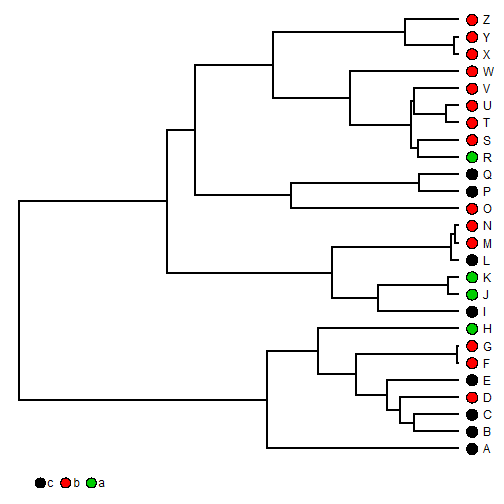Here, we'll consider six models in increasing order of parameterization: an equal-rates (`"ER"`) model, an ordered model (but with a single transition rate), an ordered symmetric model, in which `a<->b` and `b<->c` have different rates, an ordered unsymmetric model, a symmetric, un-ordered model (`"SYM"`), and an all-rates-different (`"ARD"`) model.

``````## ER model
fitER<-fitMk(tree,x,model="ER")
fitER
``````
``````## Object of class "fitMk".
##
## Fitted (or set) value of Q:
##           a         b         c
## a -0.641937  0.320969  0.320969
## b  0.320969 -0.641937  0.320969
## c  0.320969  0.320969 -0.641937
##
## Fitted (or set) value of pi:
##         a         b         c
## 0.3333333 0.3333333 0.3333333
##
## Log-likelihood: -24.08594
##
## Optimization method used was "nlminb"
``````
``````plot(fitER)
``````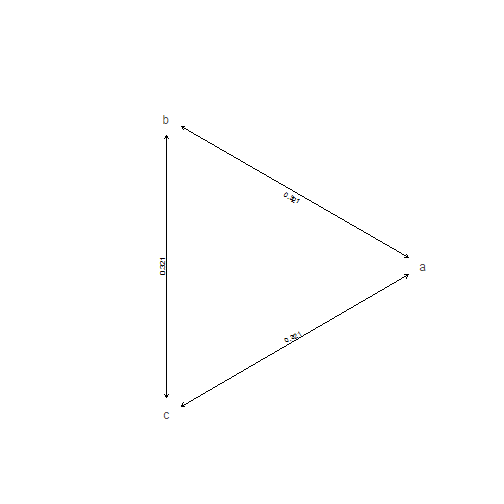``````## ordered single rate
model<-matrix(c(0,1,0,1,0,1,0,1,0),3,3,byrow=TRUE,
dimnames=list(c("a","b","c"),c("a","b","c")))
fitOrdered1<-fitMk(tree,x,model=model)
fitOrdered1
``````
``````## Object of class "fitMk".
##
## Fitted (or set) value of Q:
##           a         b         c
## a -0.685336  0.685336  0.000000
## b  0.685336 -1.370672  0.685336
## c  0.000000  0.685336 -0.685336
##
## Fitted (or set) value of pi:
##         a         b         c
## 0.3333333 0.3333333 0.3333333
##
## Log-likelihood: -24.240481
##
## Optimization method used was "nlminb"
``````
``````plot(fitOrdered1,show.zeros=FALSE)
``````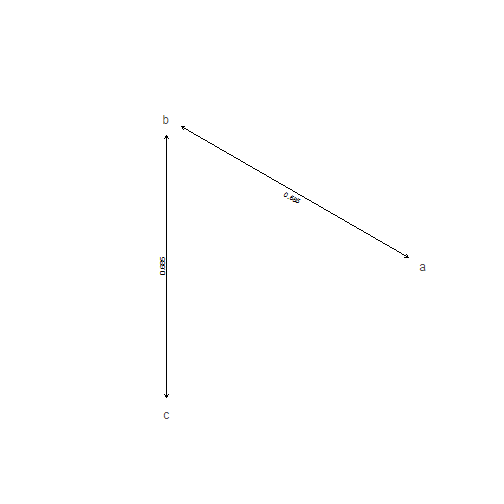``````## ordered symmetric model
model<-matrix(c(0,1,0,2,0,1,0,2,0),3,3,byrow=TRUE,
dimnames=list(c("a","b","c"),c("a","b","c")))
fitOrdered2<-fitMk(tree,x,model=model)
fitOrdered2
``````
``````## Object of class "fitMk".
##
## Fitted (or set) value of Q:
##           a         b         c
## a -0.932799  0.932799  0.000000
## b  0.688188 -1.620987  0.932799
## c  0.000000  0.688188 -0.688188
##
## Fitted (or set) value of pi:
##         a         b         c
## 0.3333333 0.3333333 0.3333333
##
## Log-likelihood: -23.890065
##
## Optimization method used was "nlminb"
``````
``````plot(fitOrdered2,show.zeros=FALSE)
``````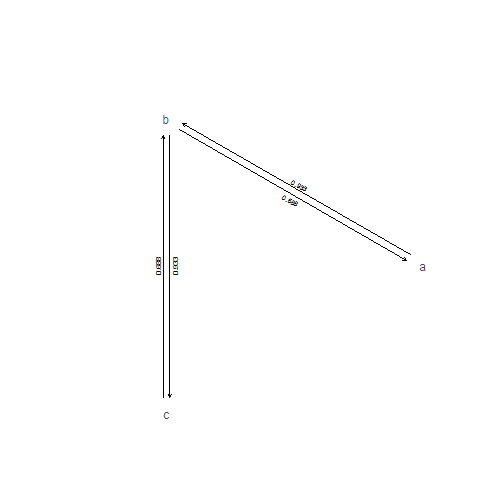``````## ordered all-rates-different model
model<-matrix(c(0,1,0,2,0,3,0,4,0),3,3,byrow=TRUE,
dimnames=list(c("a","b","c"),c("a","b","c")))
fitOrdered4<-fitMk(tree,x,model=model)
fitOrdered4
``````
``````## Object of class "fitMk".
##
## Fitted (or set) value of Q:
##           a         b         c
## a -1.724857  1.724857  0.000000
## b  0.543052 -1.145964  0.602912
## c  0.000000  0.811064 -0.811064
##
## Fitted (or set) value of pi:
##         a         b         c
## 0.3333333 0.3333333 0.3333333
##
## Log-likelihood: -22.630044
##
## Optimization method used was "nlminb"
``````
``````plot(fitOrdered4,show.zeros=FALSE)
``````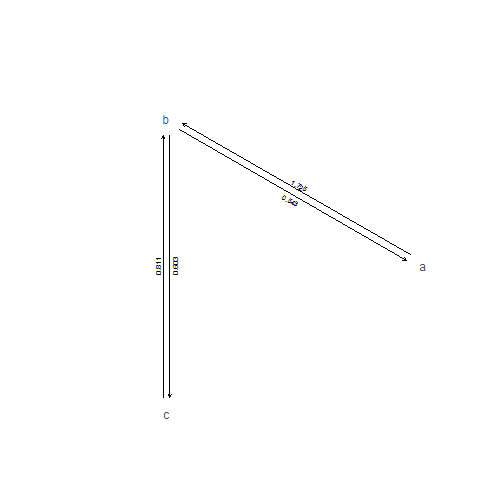``````## SYM model
fitSYM<-fitMk(tree,x,model="SYM")
fitSYM
``````
``````## Object of class "fitMk".
##
## Fitted (or set) value of Q:
##           a         b         c
## a -0.406794  0.201962  0.204833
## b  0.201962 -0.669625  0.467663
## c  0.204833  0.467663 -0.672496
##
## Fitted (or set) value of pi:
##         a         b         c
## 0.3333333 0.3333333 0.3333333
##
## Log-likelihood: -23.895651
##
## Optimization method used was "nlminb"
``````
``````plot(fitSYM,show.zeros=FALSE)
``````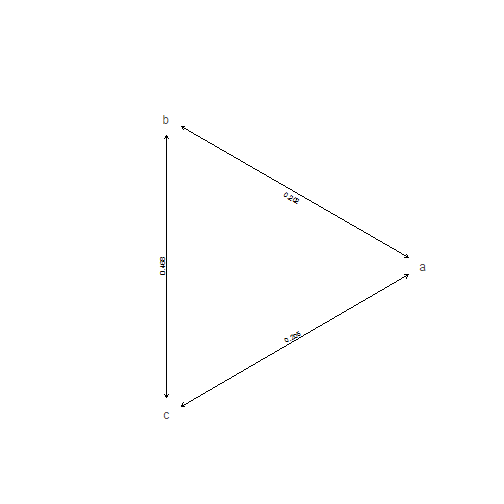``````## finally, ARD model
fitARD<-fitMk(tree,x,model="ARD")
fitARD
``````
``````## Object of class "fitMk".
##
## Fitted (or set) value of Q:
##           a         b         c
## a -0.710481  0.000000  0.710481
## b  0.282143 -0.829098  0.546955
## c  0.000000  0.989207 -0.989207
##
## Fitted (or set) value of pi:
##         a         b         c
## 0.3333333 0.3333333 0.3333333
##
## Log-likelihood: -22.681081
##
## Optimization method used was "nlminb"
``````
``````plot(fitARD,show.zeros=FALSE)
``````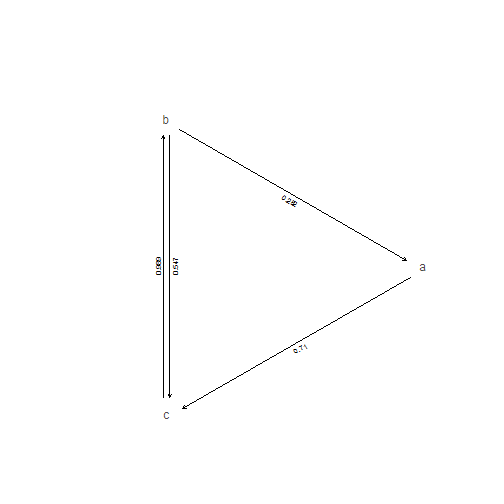Now, the question is - how can we compare among these models? Well, we can compute AICs & Akaike weights. For instance:

``````AIC<-setNames(sapply(list(fitER,fitOrdered1,fitOrdered2,fitOrdered4,fitSYM,
fitARD),AIC),c("ER","Ordered-1","Ordered-2","Ordered-4","SYM","ARD"))
AIC
``````
``````##        ER Ordered-1 Ordered-2 Ordered-4       SYM       ARD
##  50.17188  50.48096  51.78013  53.26009  53.79130  57.36216
``````
``````aic.w(AIC)
``````
``````##         ER  Ordered-1  Ordered-2  Ordered-4        SYM        ARD
## 0.36914685 0.31628807 0.16518555 0.07881405 0.06042989 0.01013560
``````

This tells us that the weight of evidence is more or less evenly split between the `"ER"` model & our single-rate ordered model.

Alternatively, we might compare pairs of models using a likelihood-ratio test. Of course, this can only be used to compare more complex models that have simpler models as a special case. This means, for instance, that we cannot compare `"ER"` and `"Ordered-1"` because they are both equally simple & neither is a special-case of the other. We can, by contrast, compare (for example) `"Ordered-1"` and `"Ordered-2"` in this way. Let's try:

``````## first the number of parameters in each model:
k0<-length(fitOrdered1\$rates)
k1<-length(fitOrdered2\$rates)
LR<--2*(logLik(fitOrdered1)-logLik(fitOrdered2))
LR
``````
``````##  0.700832
``````
``````P_chisq<-pchisq(LR,df=k1-k0,lower.tail=FALSE)
P_chisq
``````
``````##  0.4025043
``````

That's the general idea.

Note that in this simulated case `"Ordered-1"` was the generating model, so we got pretty close!